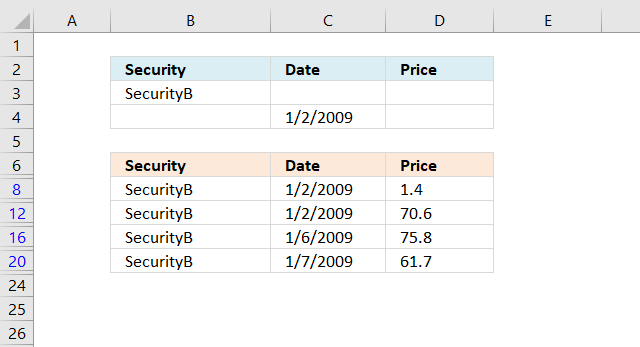Author: Oscar Cronquist Article last updated on February 24, 2019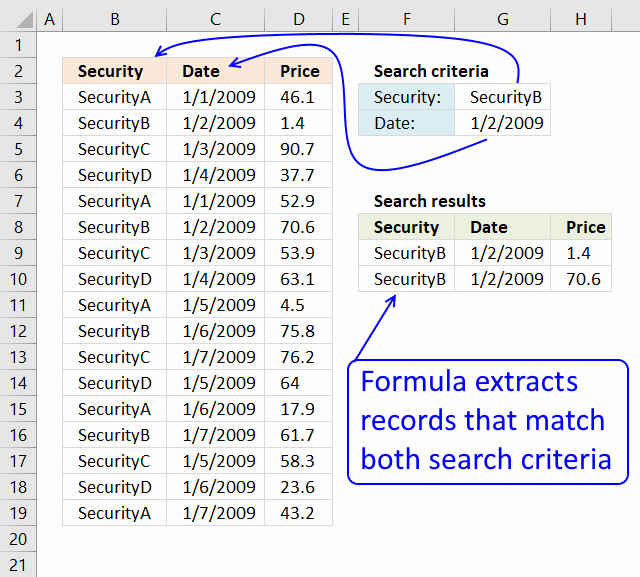Question:

I have a table of 3 columns (Security name, date, price) and I have to find the price of a security at a certain date in a table that contains many securities and prices for these securities for different dates.

If I work with vlookup or Index-match I got only the first price for certain securities. So I am not able to find the price of securities that match both the name of the securities and the date.

Could you advise if there is any way to overcome?

This article demonstrates how to extract records/rows based on two conditions applied to two different columns, you can easily extend the formula demonstrated below to include additional criteria.

If you have a scenario where you want to apply multiple conditions on a single column then read this article: Extract all rows from a range that meet criteria in one column [Array formula]

### Match two criteria and return multiple records [Array Formula]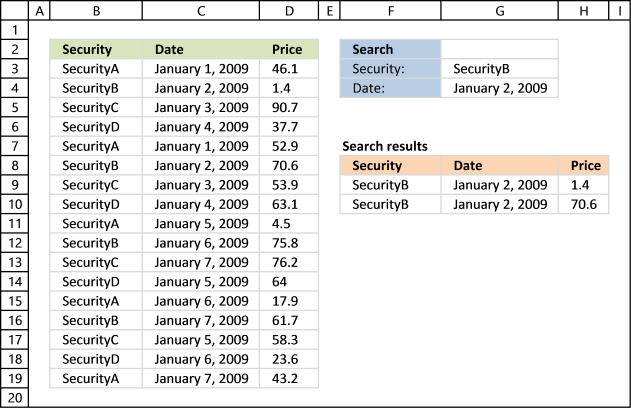The image above shows you a data set in cell range B2:D19, cell value G3 lets you match values in column B and cell G4 matches dates in column C. The formula returns matching records in cell range F9:H11 when both conditions are met.

Array formula in F9:

=INDEX(\$B\$3:\$D\$19, SMALL(IF(COUNTIF(\$G\$3, \$B\$3:\$B\$19)*COUNTIF(\$G\$4, \$C\$3:\$C\$19), ROW(\$B\$3:\$D\$19)-MIN(ROW(\$B\$3:\$D\$19))+1), ROW(A1)), COLUMN(A1))

#### How to create an array formula

1. Copy (Ctrl + c) and paste (Ctrl + v) array formula into formula bar.
2. Press and hold Ctrl + Shift.
3. Press Enter once.
4. Release all keys.

Copy cell F9 and paste it to the right. Copy cell F9:H9 and paste down as far as needed.

Alternative array formula in F9:

=INDEX(\$B\$3:\$D\$19, SMALL(IF(COUNTIFS(\$G\$3, \$B\$3:\$B\$19, \$G\$4, \$C\$3:\$C\$19), ROW(\$B\$3:\$D\$19)-MIN(ROW(\$B\$3:\$D\$19))+1), ROW(A1)), COLUMN(A1))

### Explaining excel array formula in cell range F9:H10

#### Step 1 - Identify matching cell values

=INDEX(tbl, SMALL(IF(COUNTIF(\$G\$3, \$B\$3:\$B\$19)*COUNTIF(\$G\$4, \$C\$3:\$C\$19), ROW(tbl)-MIN(ROW(tbl))+1), ROW(A1)), COLUMN(A1))

COUNTIF(\$G\$3, \$B\$3:\$B\$19)

becomes

COUNTIF("SecurityB", {SecurityA, SecurityB, SecurityC, SecurityD, SecurityA, SecurityB, SecurityC, SecurityD, SecurityA, SecurityB, SecurityC, SecurityD, SecurityA, SecurityB, SecurityC, SecurityD, SecurityA})

and reurns this array: {0, 1, 0, 0, 0, 1, 0, 0, 0, 1, 0, 0, 0, 1, 0, 0, 0}

COUNTIF(\$G\$4, \$C\$3:\$C\$19) returns this array: {0, 1, 0, 0, 0, 1, 0, 0, 0, 0, 0, 0, 0, 0, 0, 0, 0}

COUNTIF(\$G\$3, \$B\$3:\$B\$19)*COUNTIF(\$G\$4, \$C\$3:\$C\$19) becomes {0, 1, 0, 0, 0, 1, 0, 0, 0, 1, 0, 0, 0, 1, 0, 0, 0}*{0, 1, 0, 0, 0, 1, 0, 0, 0, 0, 0, 0, 0, 0, 0, 0, 0} and returns: {0, 1, 0, 0, 0, 1, 0, 0, 0, 0, 0, 0, 0, 0, 0, 0, 0}

How to use the COUNTIF function

Counts the number of cells that meet a specific condition.

#### Step 2 - Match array to row number

IF(COUNTIF(\$G\$3, \$B\$3:\$B\$19)*COUNTIF(\$G\$4, \$C\$3:\$C\$19), ROW(tbl)-MIN(ROW(tbl))+1)

becomes

IF({0, 1, 0, 0, 0, 1, 0, 0, 0, 0, 0, 0, 0, 0, 0, 0, 0}, 1, 2, 3, 4, 5, 6, 7, 8, 9, 10, 11, 12, 13, 14, 15, 16, 17)

and returns:

{False, 2, False, False, False, 6, False, False, False, False, False, False, False, False, False, False, False}

How to use the IF function

Checks if a logical expression is met. Returns a specific value if TRUE and another specific value if FALSE.

#### Step 3 - Get table value using row number

Array formula in cell F9:

=INDEX(tbl, SMALL(IF(COUNTIF(\$G\$3, \$B\$3:\$B\$19)*COUNTIF(\$G\$4, \$C\$3:\$C\$19), ROW(tbl)-MIN(ROW(tbl))+1), ROW(A1)), COLUMN(A1))

becomes

=INDEX(tbl, SMALL({False, 2, False, False, False, 6, False, False, False, False, False, False, False, False, False, False, False}, ROW(A1)), COLUMN(A1))

becomes

=INDEX(tbl, SMALL({False, 2, False, False, False, 6, False, False, False, False, False, False, False, False, False, False, False}, 1), COLUMN(A1))

becomes

=INDEX(tbl, 2, 1) and returns "Security B"

How to use the INDEX function

Gets a value in a specific cell range based on a row and column number.

* You will also get a weekly newsletter, unsubscribe anytime!

#### Recommended articles

The following post shows you how to filter records using a single condition:
VLOOKUP - return multiple records

### Match two criteria and return multiple records [Excel defined Table]

1. Select the range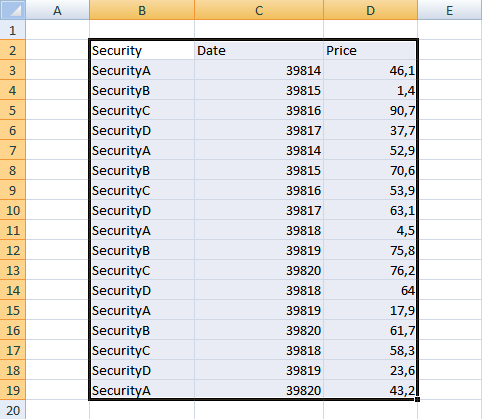2. Click "Insert" tab
3. Click "Table"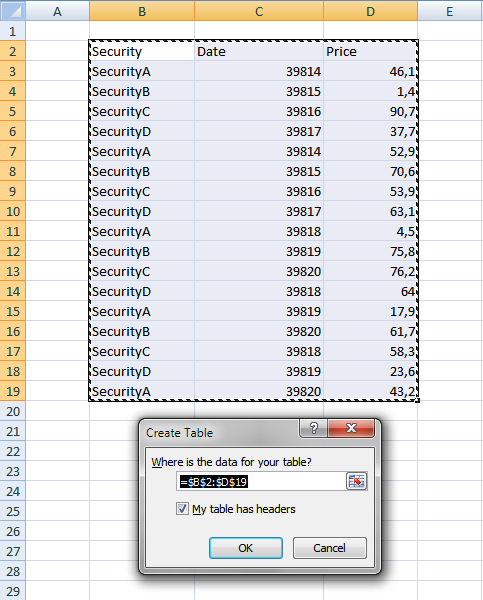4. Click OK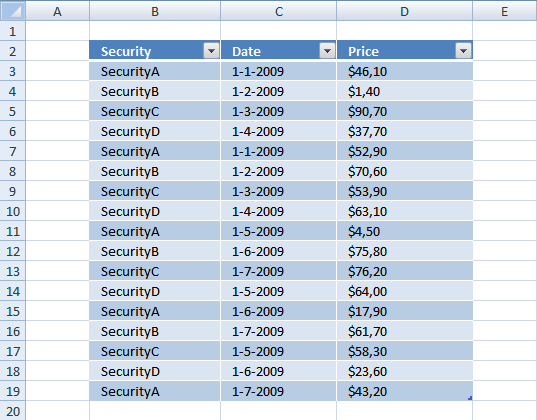1. Click black arrow next to header "Security".
2. Select the items you want to filter.
3. Click black arrow next to header "Date".
4. Make sure only 1-2-2009 is selected.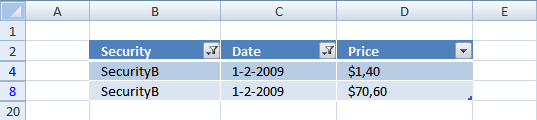The image above shows both conditions applied to the Excel defined Table.

### Match two criteria and return multiple records [Advanced Filter]The image above demonstrates a filter applied to a data set using Excel's Advanced Filter feature. Here is how to create that filter:

1. Copy headers and paste to cells below or above the dataset.
Note, the filter values may become hidden if you place them next to the dataset.
2. Type the conditions below each header accordingly.
3. Select the dataset.
4. Go to tab "Data" on the ribbon.
5. Click "Advanced" button, a dialog box appears.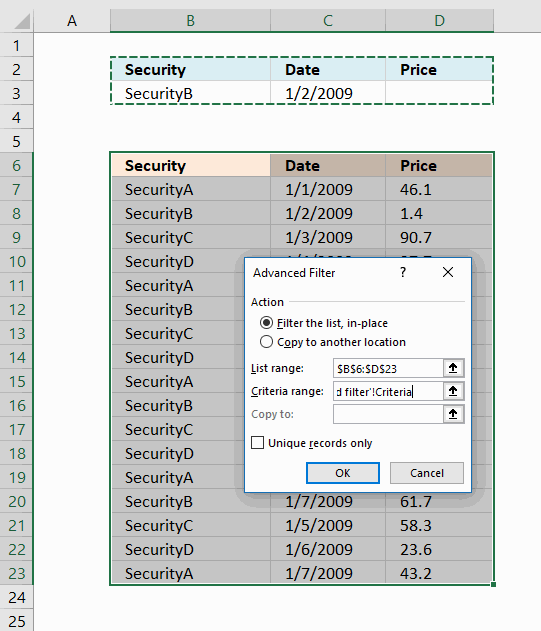6. Click radio button "Filter the list, in place".
7. Click "Criteria range:" field and select cell range B2:D3, see image above.
8. Click OK button.The image above shows records filtered on items based on condition in B3 and dates based on condition in C3. If both conditions match on the same row the record/row appears in the filtered list.

Put the conditions on a row each in order to apply OR-logic instead of AND-logic between conditions, see image below.